# RD Sharma Solutions for Class 10 Maths Chapter 9 Arithmetic Progressions Exercise 9.4

The concepts of the general term, nth term from the end and middle term of an A.P are testified in this exercise. As these are the main parts of the chapter, we strongly recommend students to refer the RD Sharma Solutions Class 10 prepared by subject experts at BYJU’S as its a very useful resource for students to prepare well for their exams. Students can use RD Sharma Solutions for Class 10 Maths Chapter 9 Arithmetic Progressions Exercise 9.4 PDF for getting a clear understanding of the problems.

## RD Sharma Solutions for Class 10 Maths Chapter 9 Arithmetic Progressions Exercise 9.4 Download PDF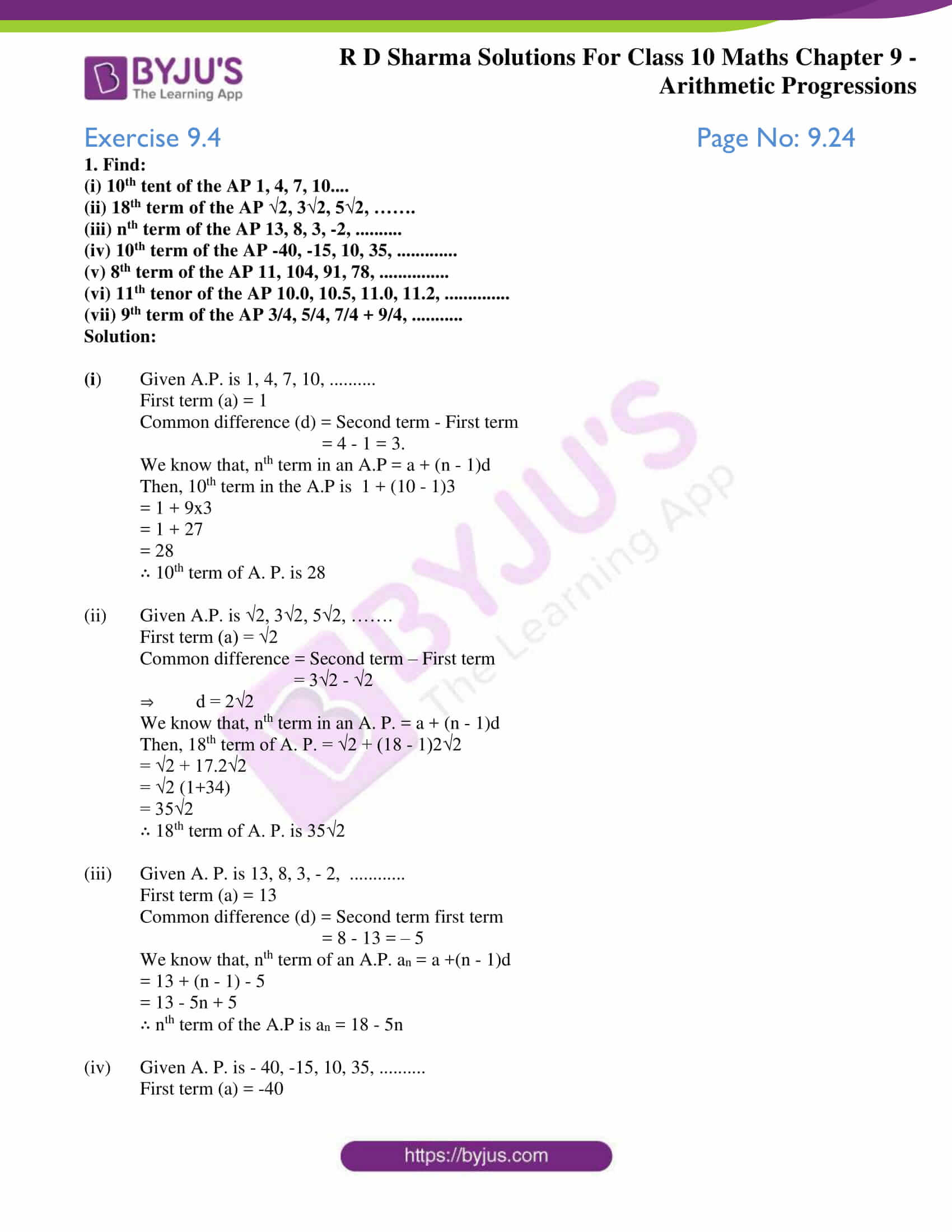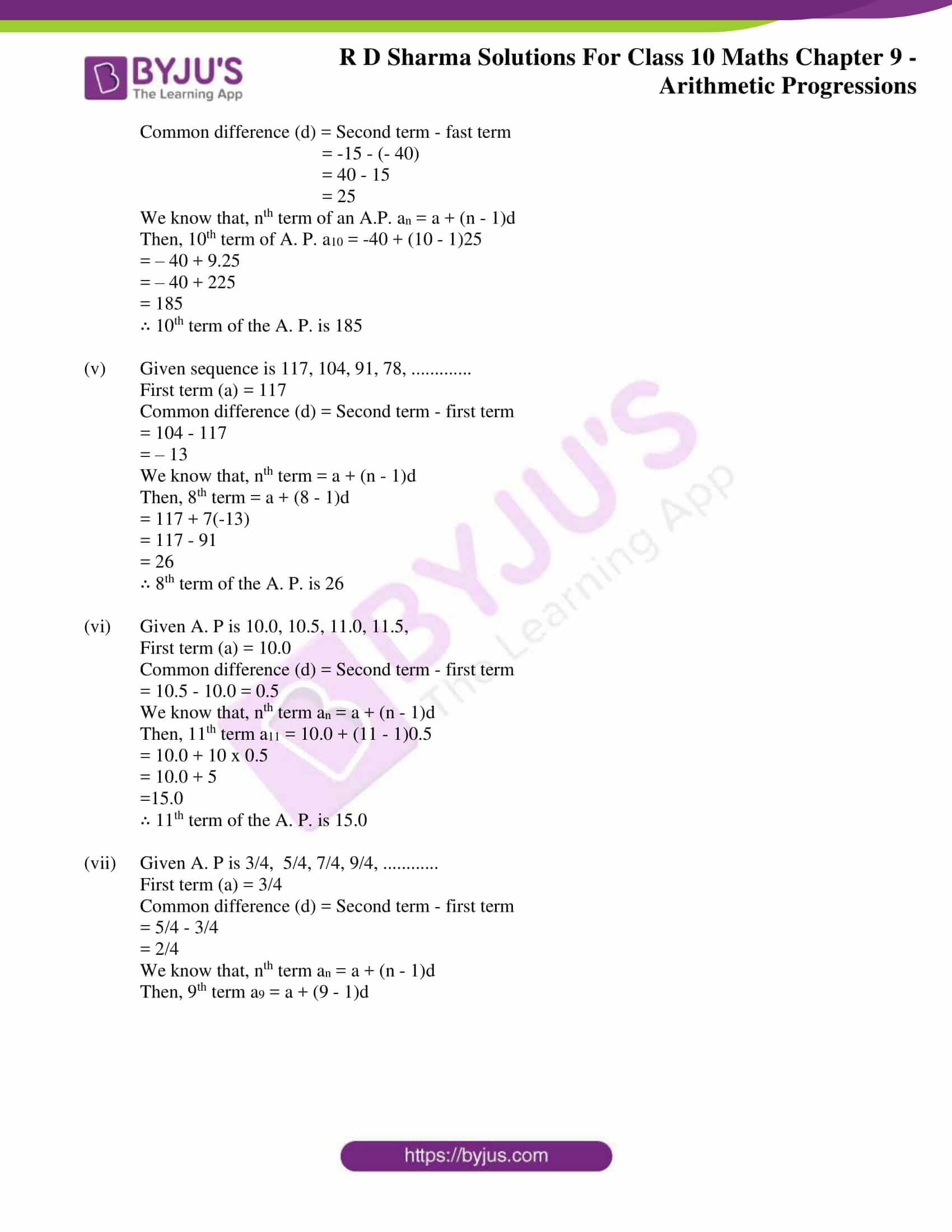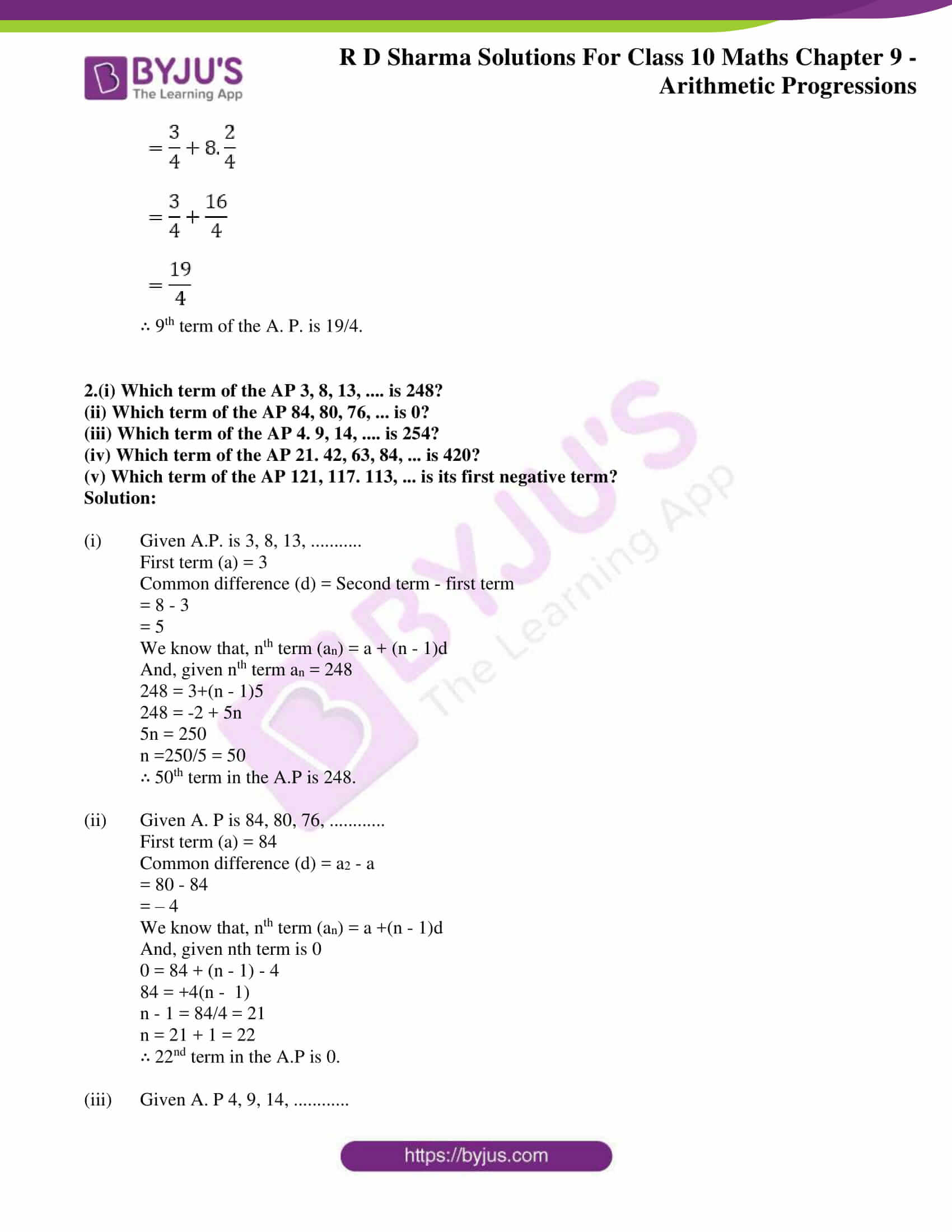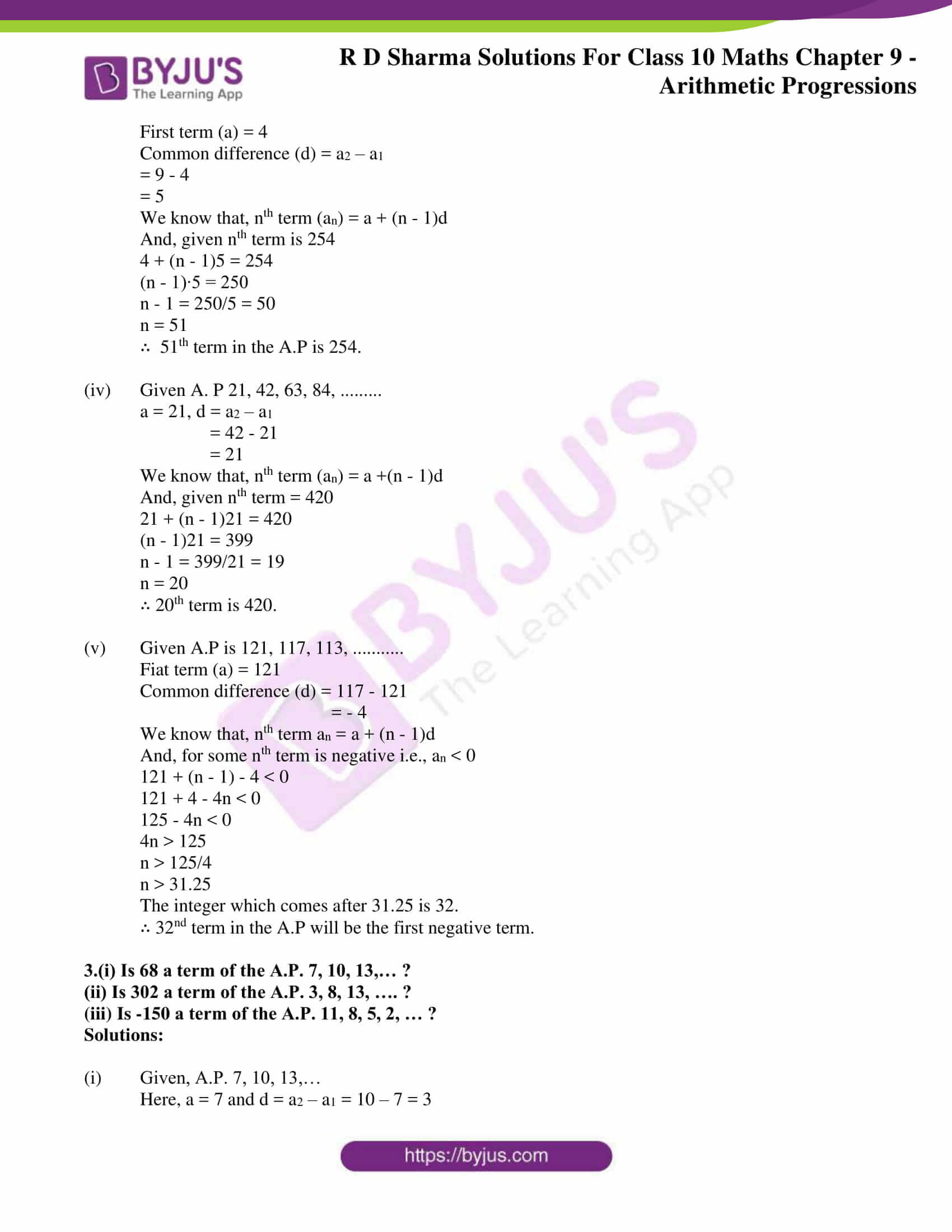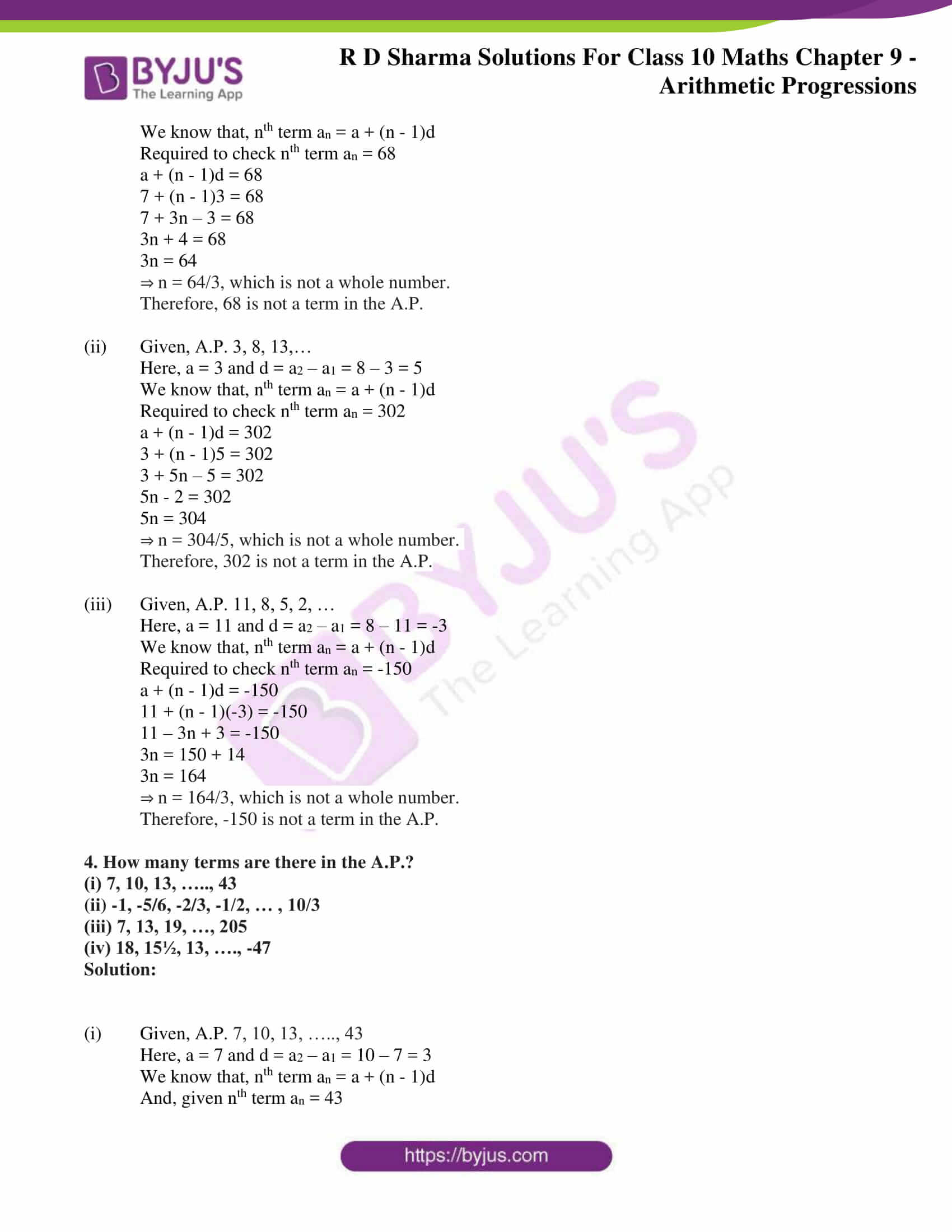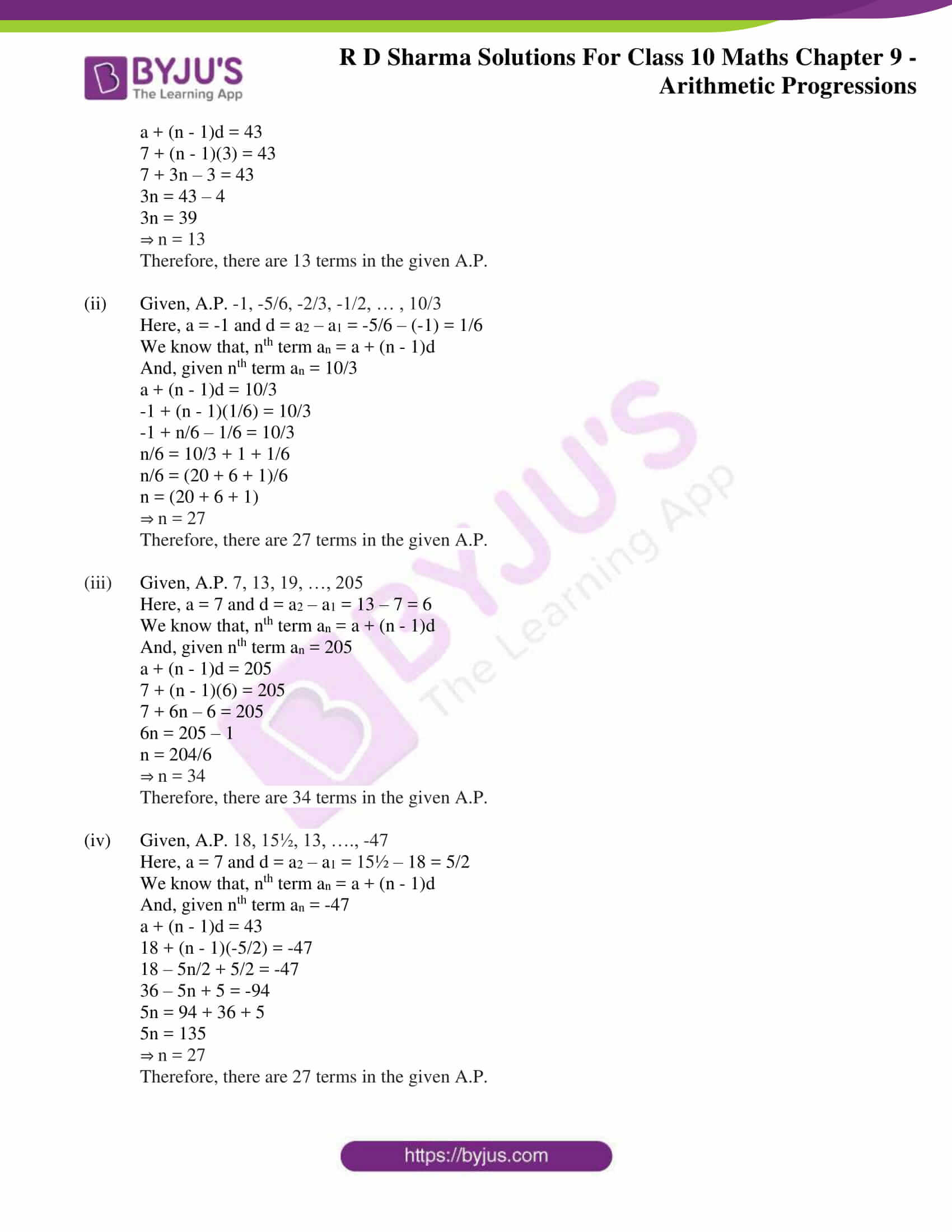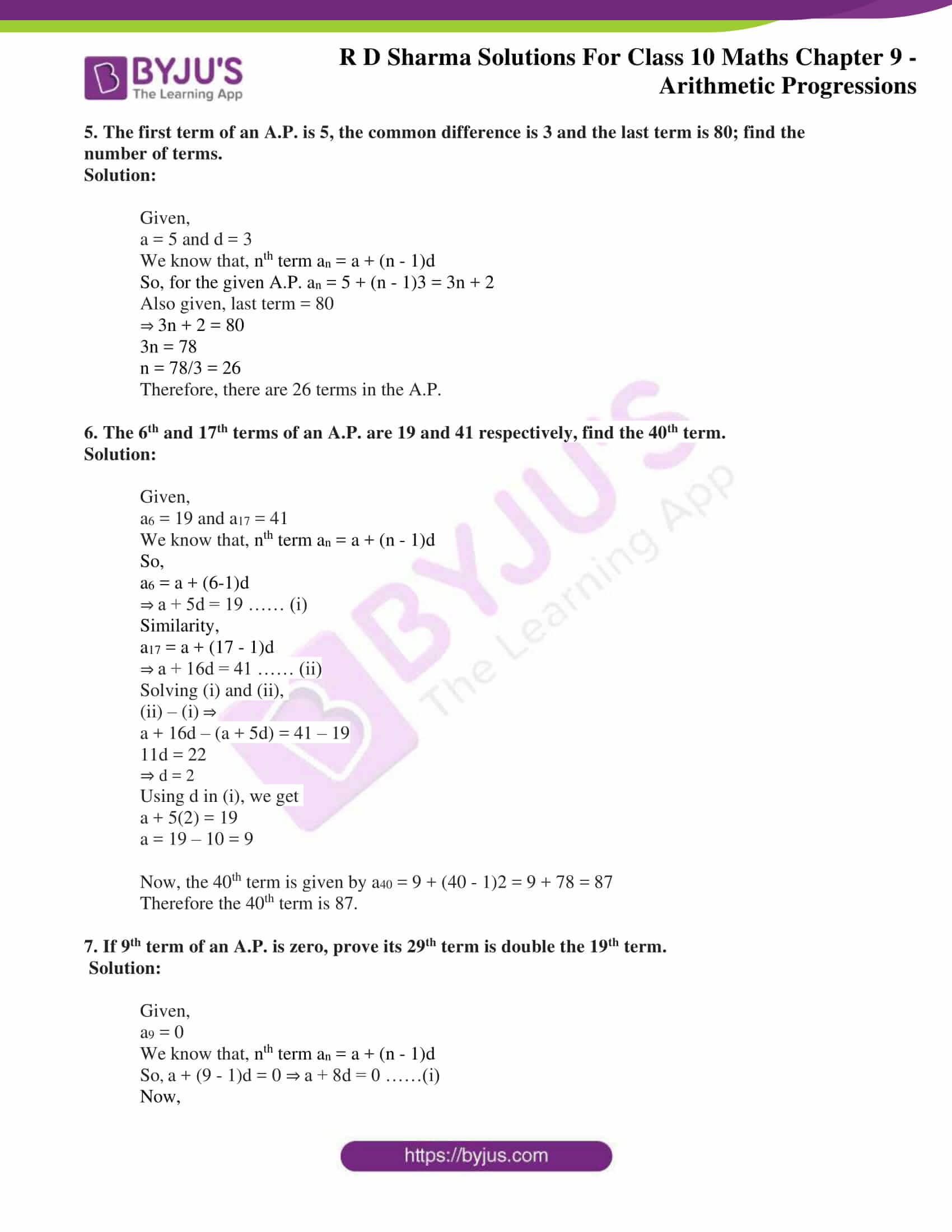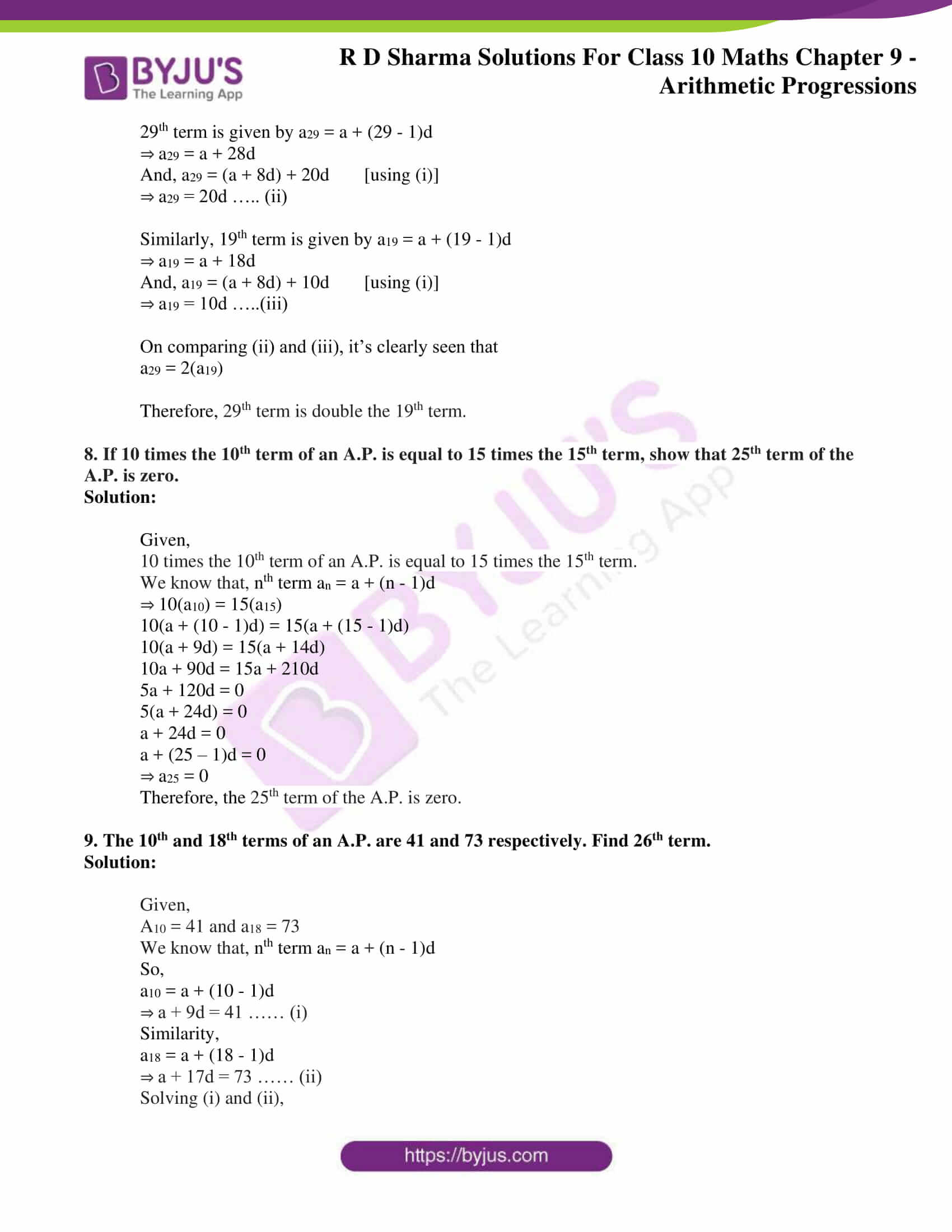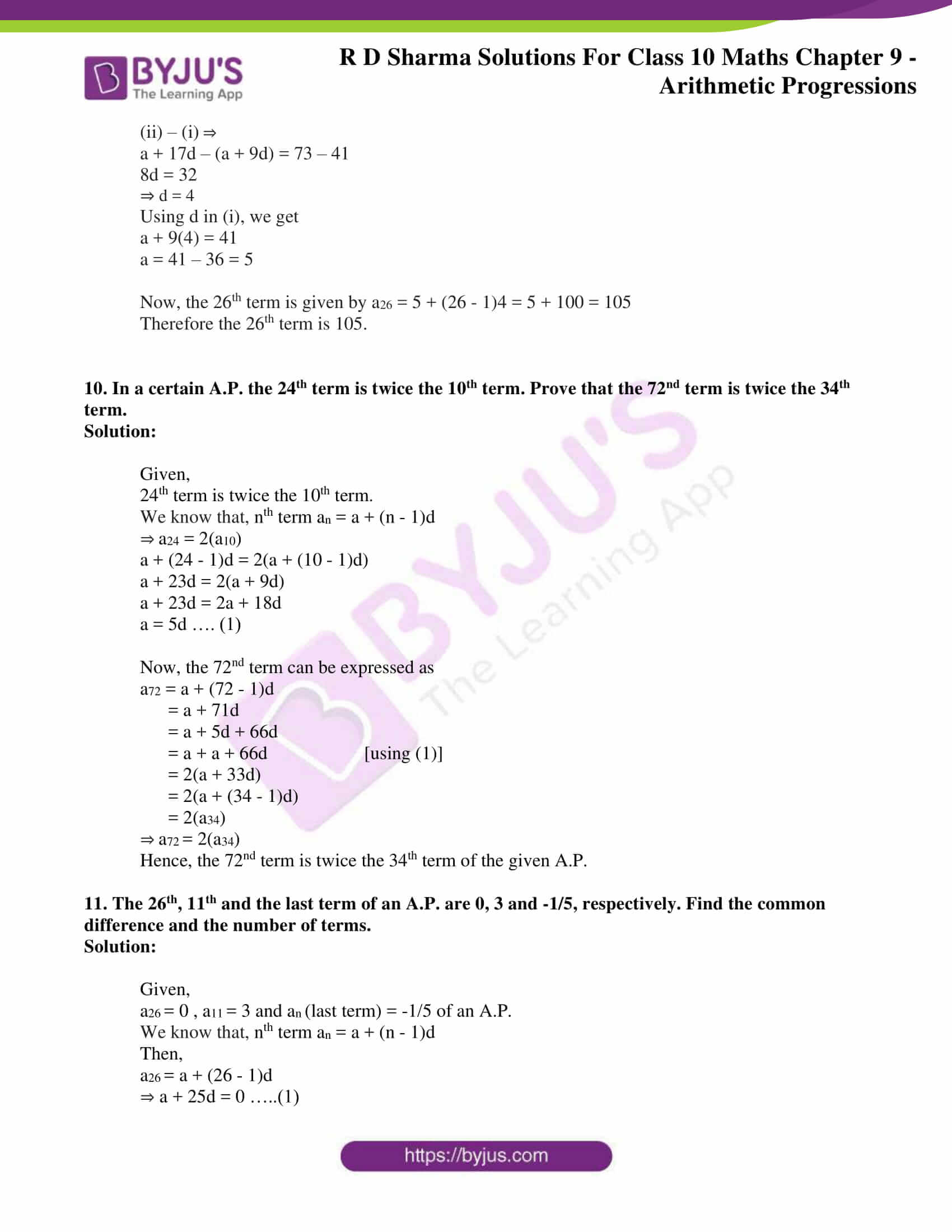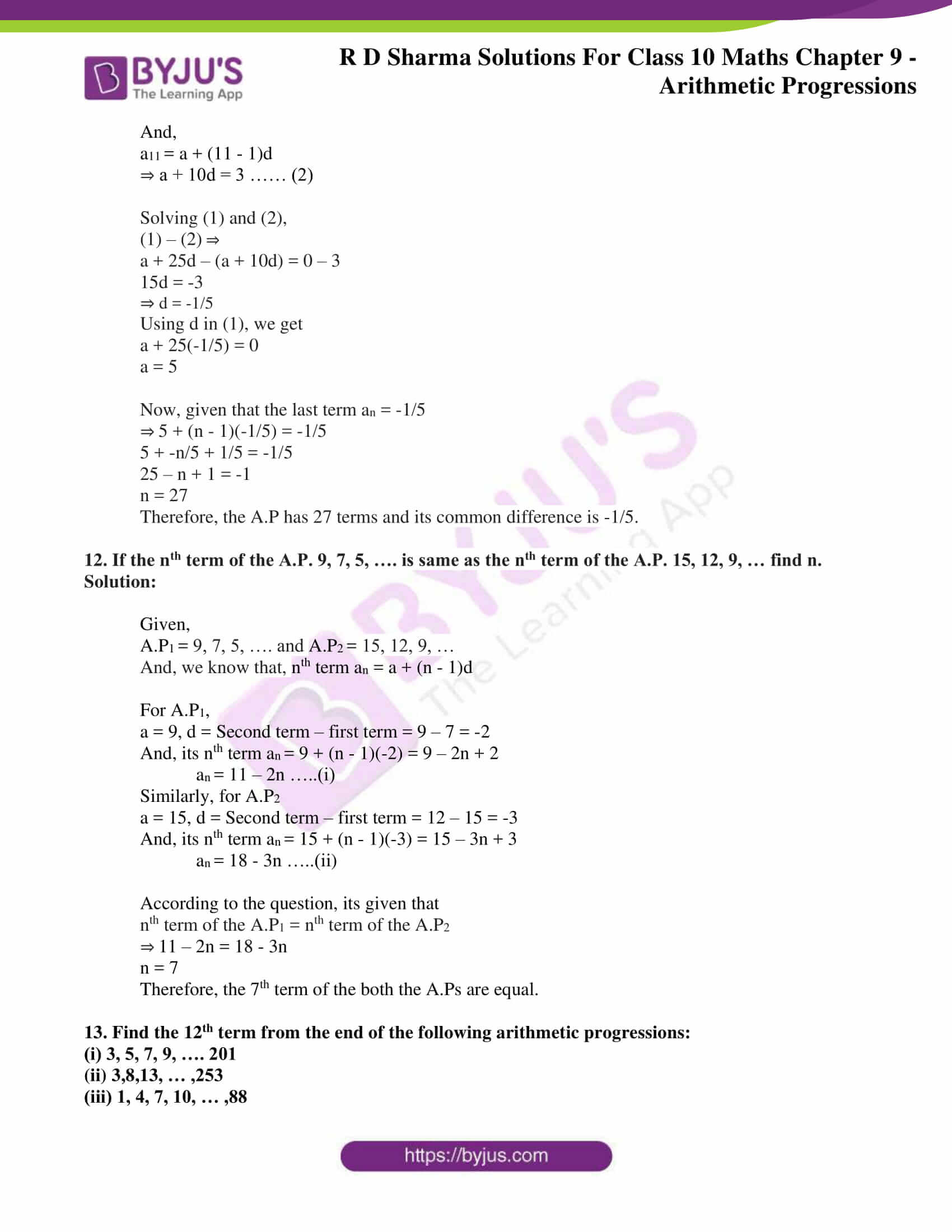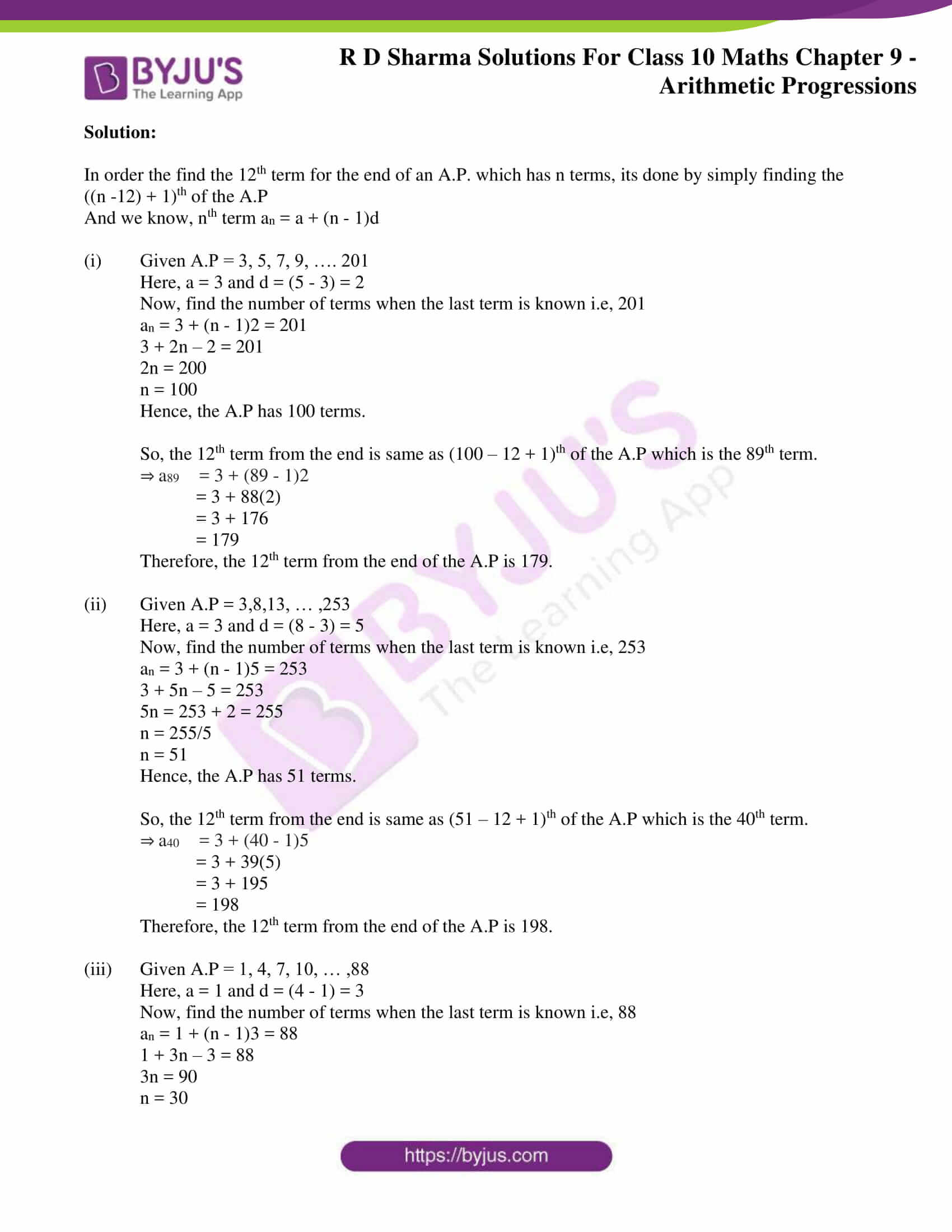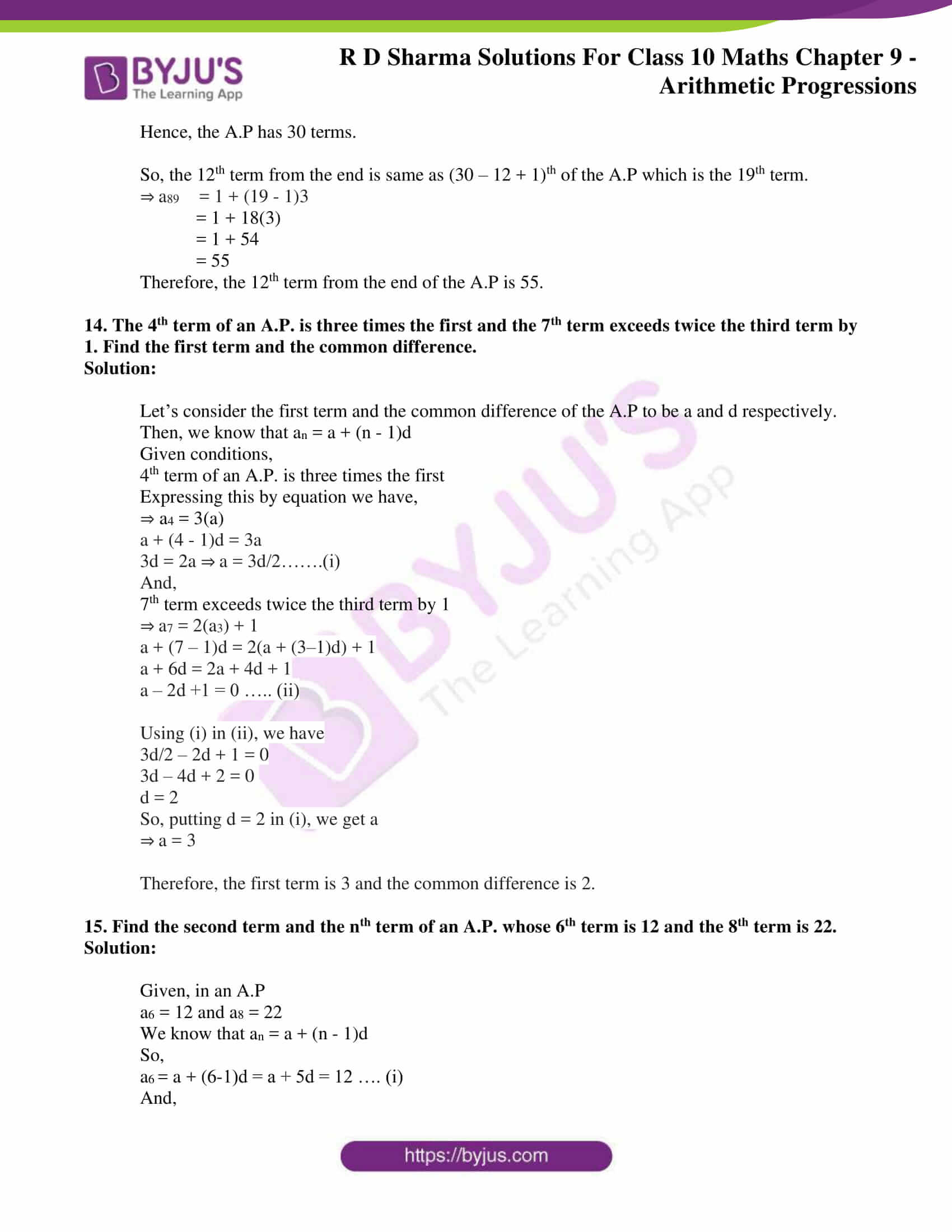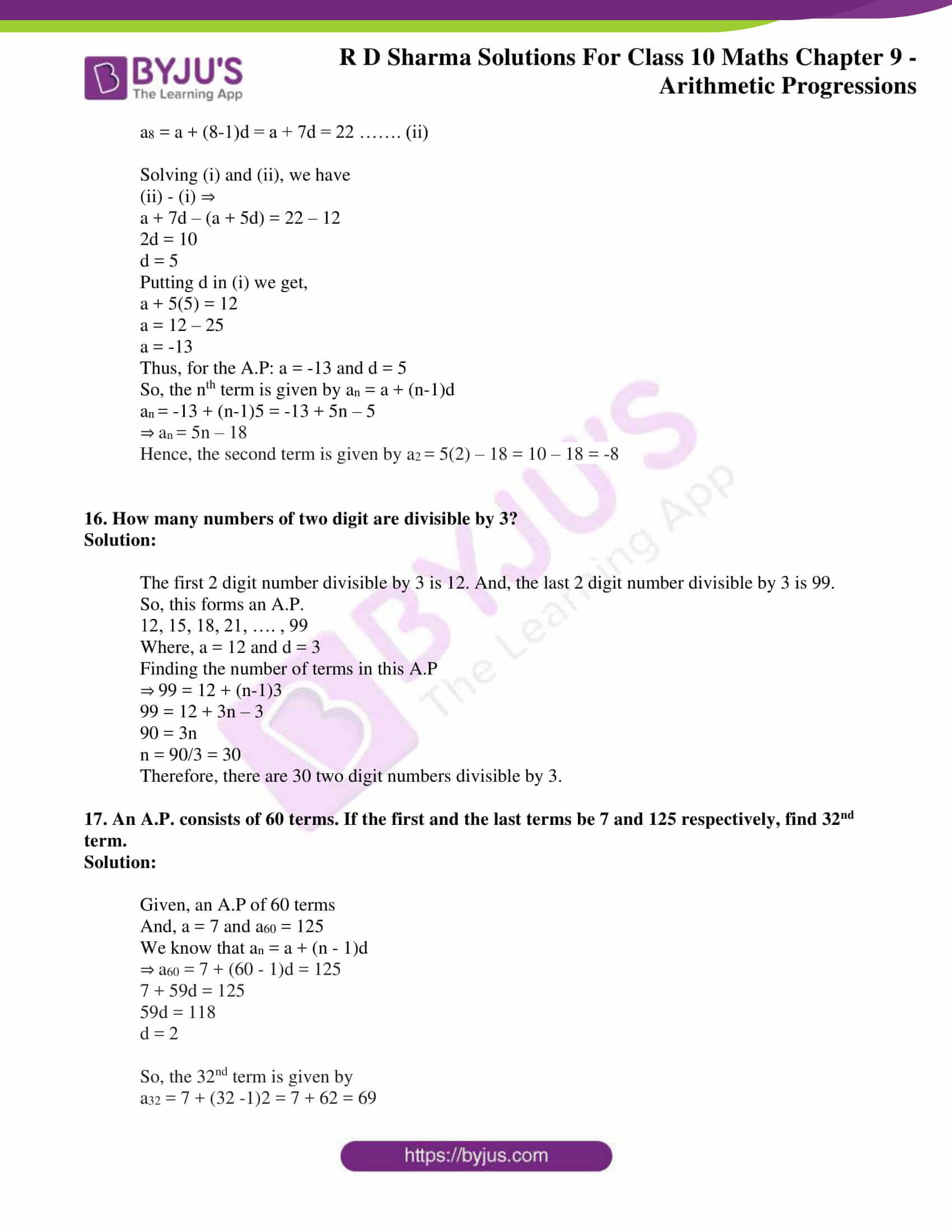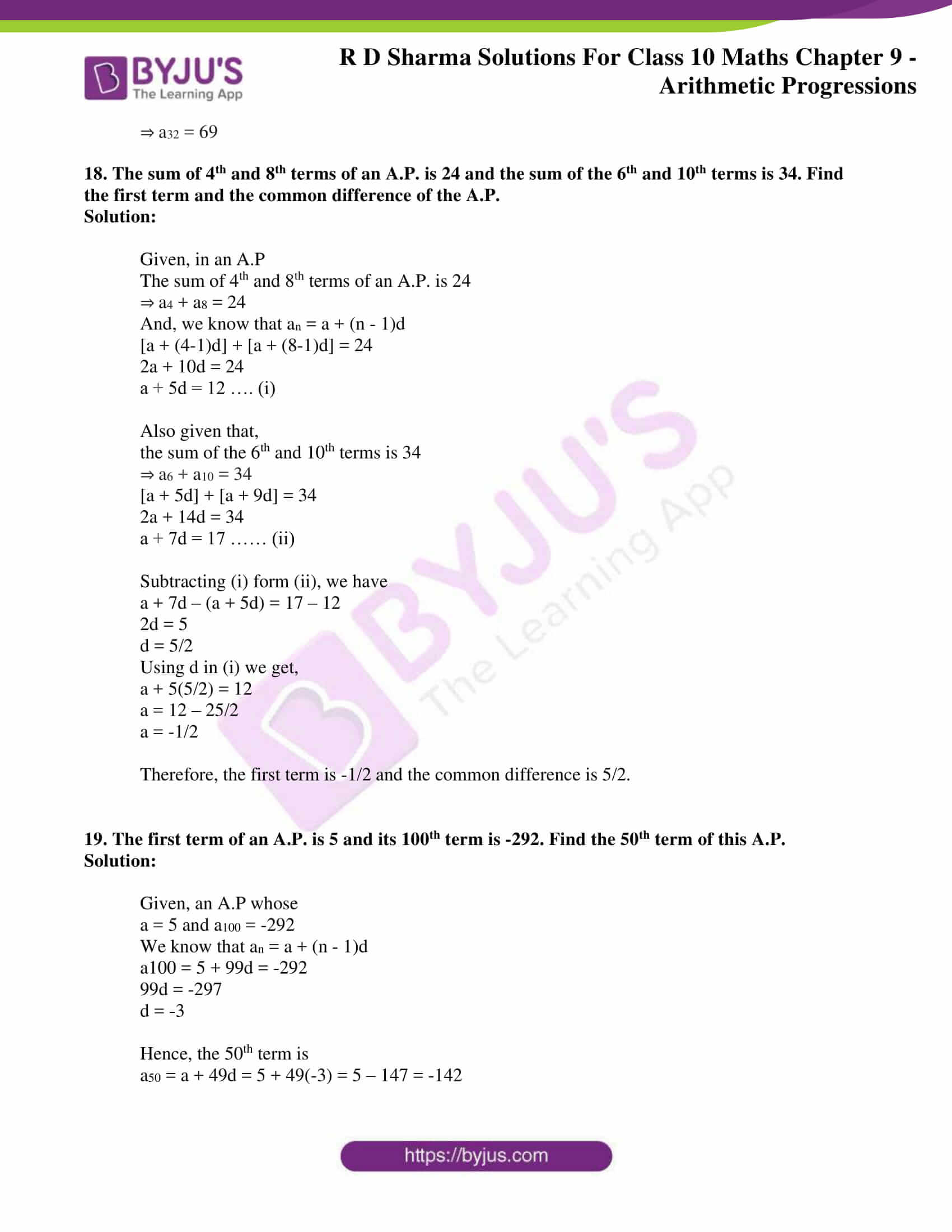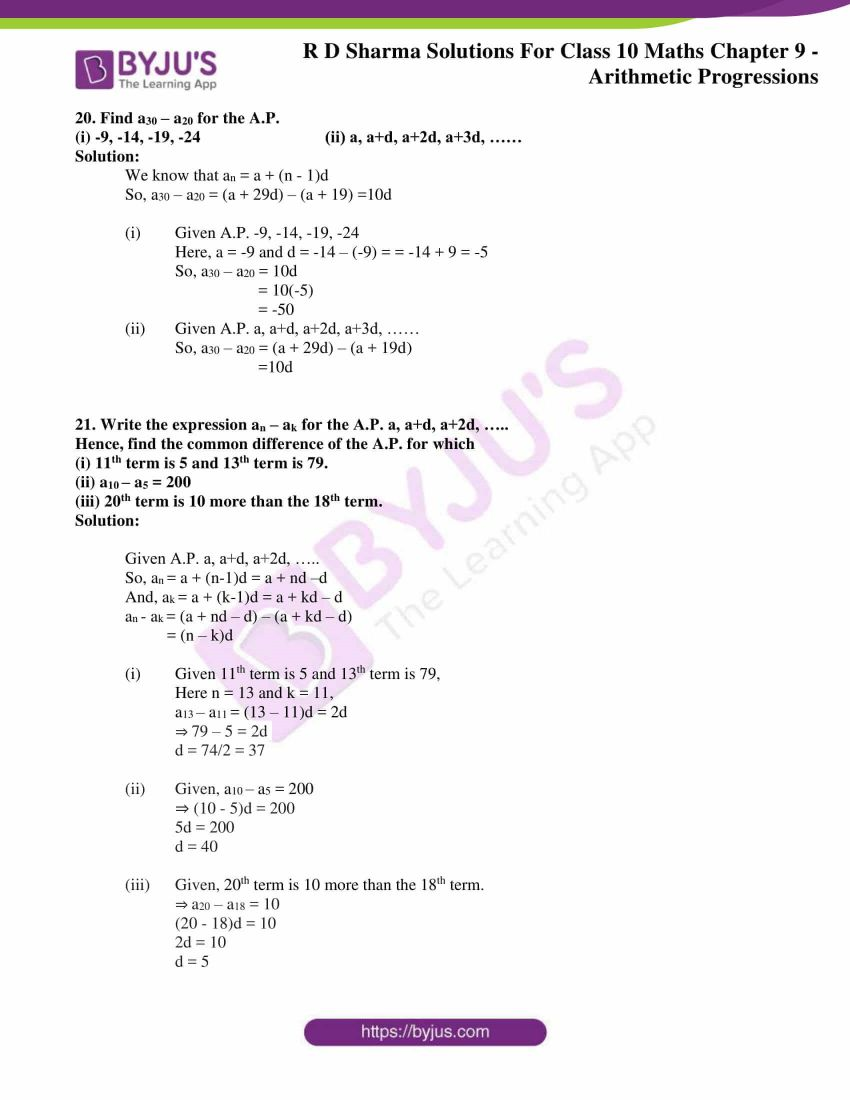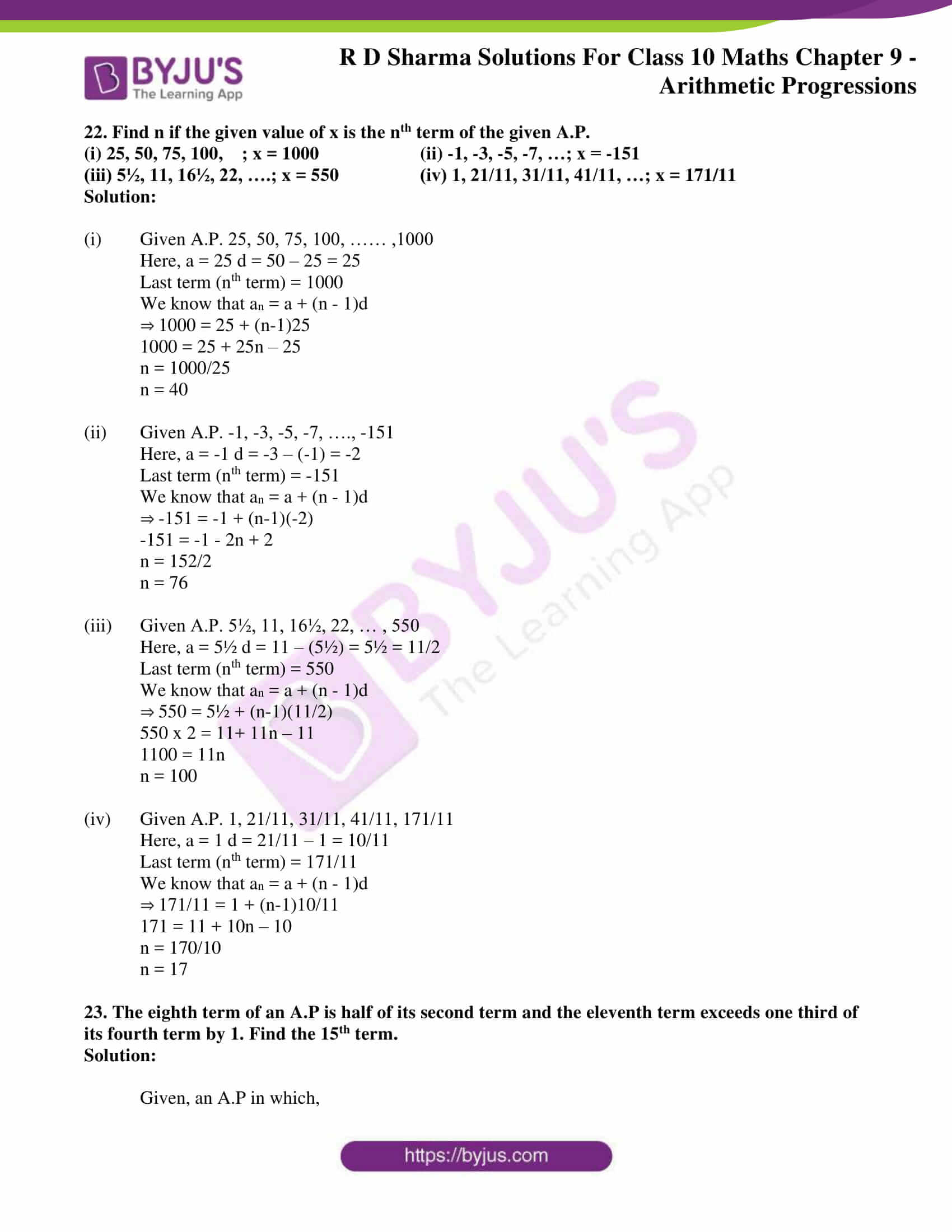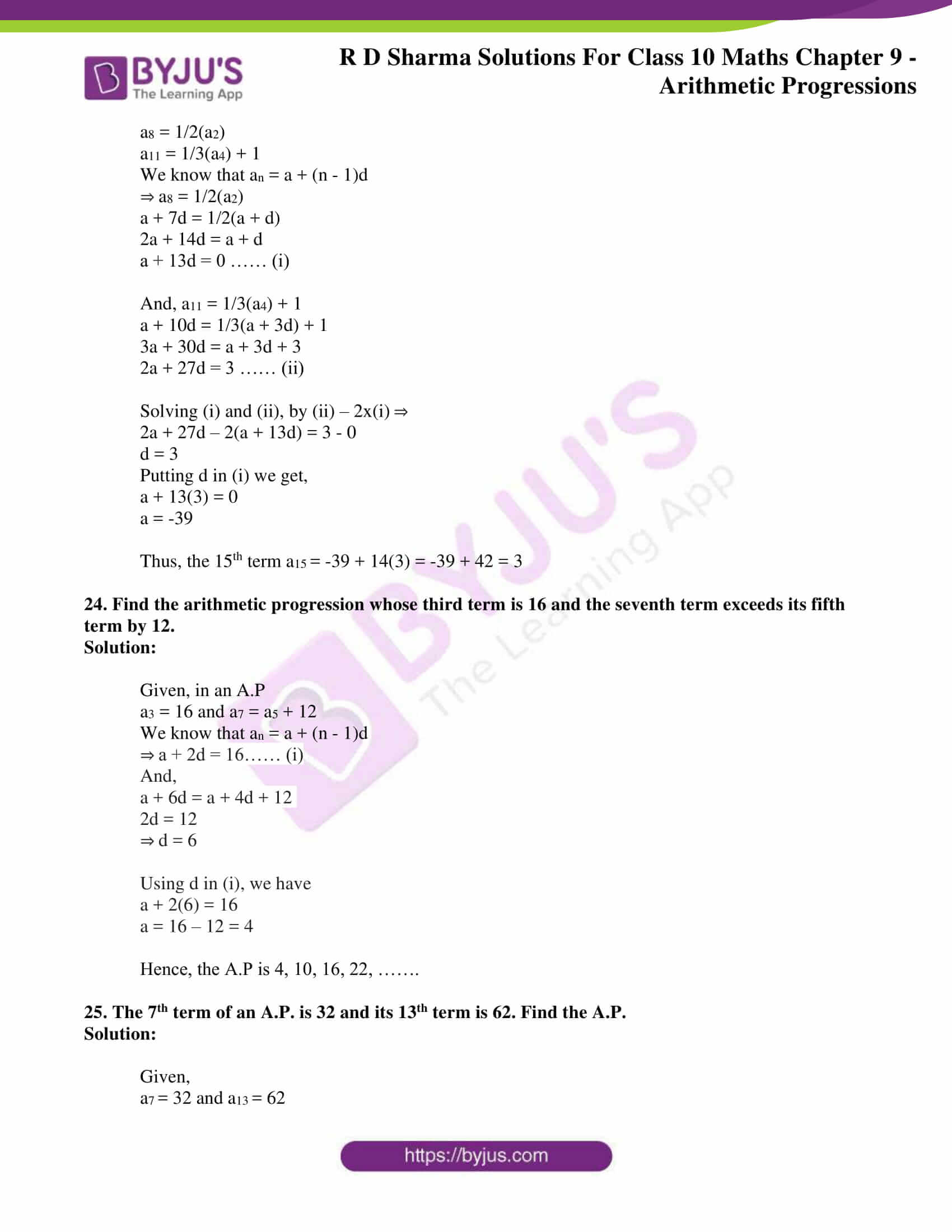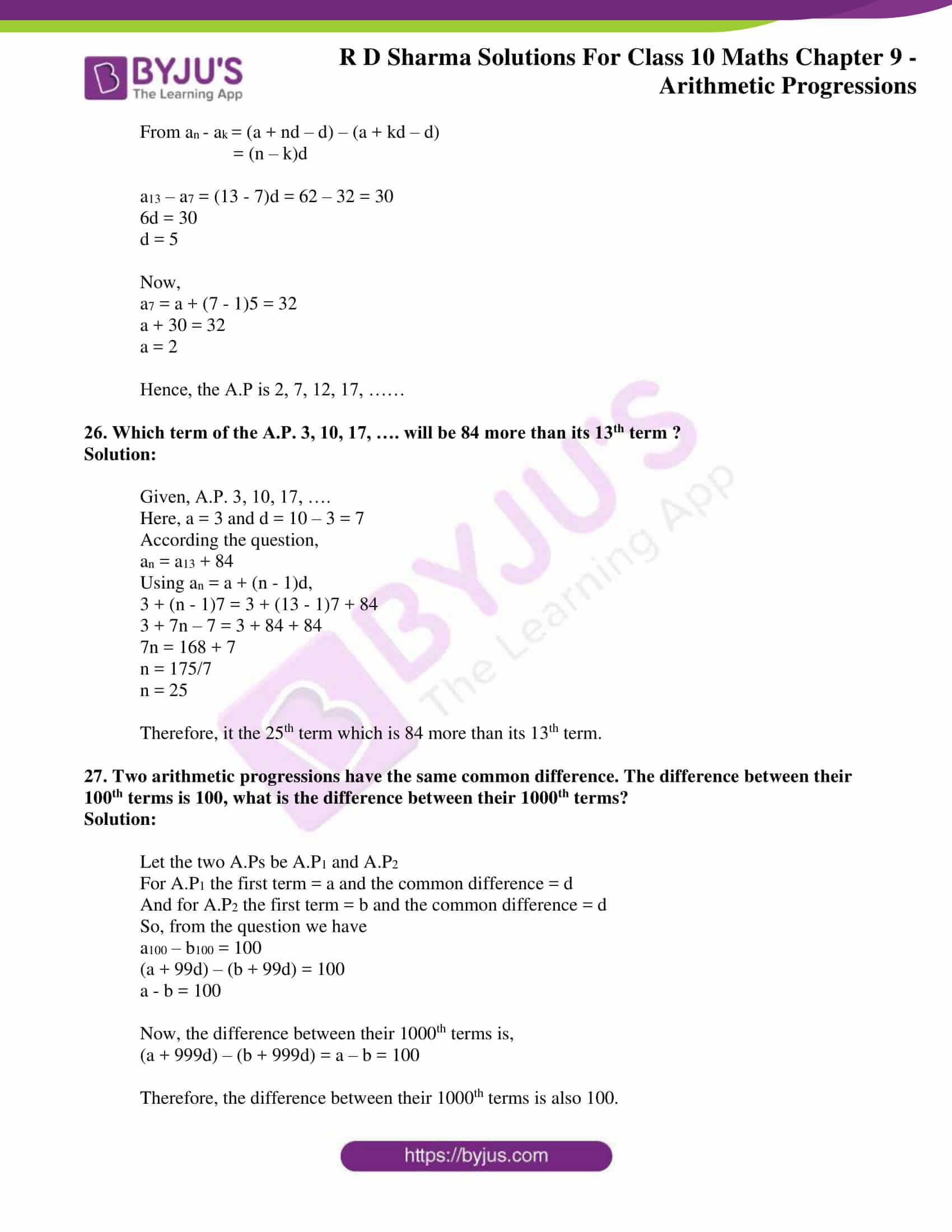### Access RD Sharma Solutions for Class 10 Maths Chapter 9 Arithmetic Progressions Exercise 9.4

1. Find:

(i) 10th tent of the AP 1, 4, 7, 10….

(ii) 18th term of the AP √2, 3√2, 5√2, …….

(iii) nth term of the AP 13, 8, 3, -2, ……….

(iv) 10th term of the AP -40, -15, 10, 35, ………….

(v) 8th term of the AP 11, 104, 91, 78, ……………

(vi) 11th tenor of the AP 10.0, 10.5, 11.0, 11.2, …………..

(vii) 9th term of the AP 3/4, 5/4, 7/4 + 9/4, ………..

Solution:

(i) Given A.P. is 1, 4, 7, 10, ……….

First term (a) = 1

Common difference (d) = Second term – First term

= 4 – 1 = 3.

We know that, nth term in an A.P = a + (n – 1)d

Then, 10th term in the A.P is 1 + (10 – 1)3

= 1 + 9×3

= 1 + 27

= 28

∴ 10th term of A. P. is 28

(ii) Given A.P. is √2, 3√2, 5√2, …….

First term (a) = √2

Common difference = Second term – First term

= 3√2 – √2

⇒ d = 2√2

We know that, nth term in an A. P. = a + (n – 1)d

Then, 18th term of A. P. = √2 + (18 – 1)2√2

= √2 + 17.2√2

= √2 (1+34)

= 35√2

∴ 18th term of A. P. is 35√2

(iii) Given A. P. is 13, 8, 3, – 2,  …………

First term (a) = 13

Common difference (d) = Second term first term

= 8 – 13 = – 5

We know that, nth term of an A.P. an = a +(n – 1)d

= 13 + (n – 1) – 5

= 13 – 5n + 5

∴ nth term of the A.P is an = 18 – 5n

(iv) Given A. P. is – 40, -15, 10, 35, ……….

First term (a) = -40

Common difference (d) = Second term – fast term

= -15 – (- 40)

= 40 – 15

= 25

We know that, nth term of an A.P. an = a + (n – 1)d

Then, 10th term of A. P. a10 = -40 + (10 – 1)25

= – 40 + 9.25

= – 40 + 225

= 185

∴ 10th term of the A. P. is 185

(v) Given sequence is 117, 104, 91, 78, ………….

First term (a) = 117

Common difference (d) = Second term – first term

= 104 – 117

= – 13

We know that, nth term = a + (n – 1)d

Then, 8th term = a + (8 – 1)d

= 117 + 7(-13)

= 117 – 91

= 26

∴ 8th term of the A. P. is 26

(vi) Given A. P is 10.0, 10.5, 11.0, 11.5,

First term (a) = 10.0

Common difference (d) = Second term – first term

= 10.5 – 10.0 = 0.5

We know that, nth term an = a + (n – 1)d

Then, 11th term a11 = 10.0 + (11 – 1)0.5

= 10.0 + 10 x 0.5

= 10.0 + 5

=15.0

∴ 11th term of the A. P. is 15.0

(vii) Given A. P is 3/4,  5/4, 7/4, 9/4, …………

First term (a) = 3/4

Common difference (d) = Second term – first term

= 5/4 – 3/4

= 2/4

We know that, nth term an = a + (n – 1)d

Then, 9th term a9 = a + (9 – 1)d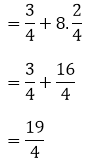∴ 9th term of the A. P. is 19/4.

2.(i) Which term of the AP 3, 8, 13, …. is 248?

(ii) Which term of the AP 84, 80, 76, … is 0?

(iii) Which term of the AP 4. 9, 14, …. is 254?

(iv) Which term of the AP 21. 42, 63, 84, … is 420?

(v) Which term of the AP 121, 117. 113, … is its first negative term?

Solution:

(i) Given A.P. is 3, 8, 13, ………..

First term (a) = 3

Common difference (d) = Second term – first term

= 8 – 3

= 5

We know that, nth term (an) = a + (n – 1)d

And, given nth term an = 248

248 = 3+(n – 1)5

248 = -2 + 5n

5n = 250

n =250/5 = 50

∴ 50th term in the A.P is 248.

(ii) Given A. P is 84, 80, 76, …………

First term (a) = 84

Common difference (d) = a2 – a

= 80 – 84

= – 4

We know that, nth term (an) = a +(n – 1)d

And, given nth term is 0

0 = 84 + (n – 1) – 4

84 = +4(n –  1)

n – 1 = 84/4 = 21

n = 21 + 1 = 22

∴ 22nd term in the A.P is 0.

(iii) Given A. P 4, 9, 14, …………

First term (a) = 4

Common difference (d) = a2 – a1

= 9 – 4

= 5

We know that, nth term (an) = a + (n – 1)d

And, given nth term is 254

4 + (n – 1)5 = 254

(n – 1)∙5 = 250

n – 1 = 250/5 = 50

n = 51

∴  51th term in the A.P is 254.

(iv) Given A. P 21, 42, 63, 84, ………

a = 21, d = a2 – a1

= 42 – 21

= 21

We know that, nth term (an) = a +(n – 1)d

And, given nth term = 420

21 + (n – 1)21 = 420

(n – 1)21 = 399

n – 1 = 399/21 = 19

n = 20

∴ 20th term is 420.

(v) Given A.P is 121, 117, 113, ………..

Fiat term (a) = 121

Common difference (d) = 117 – 121

= – 4

We know that, nth term an = a + (n – 1)d

And, for some nth term is negative i.e., an < 0

121 + (n – 1) – 4 < 0

121 + 4 – 4n < 0

125 – 4n < 0

4n > 125

n > 125/4

n > 31.25

The integer which comes after 31.25 is 32.

∴ 32nd term in the A.P will be the first negative term.

3.(i) Is 68 a term of the A.P. 7, 10, 13,… ?

(ii) Is 302 a term of the A.P. 3, 8, 13, …. ?

(iii) Is -150 a term of the A.P. 11, 8, 5, 2, … ?

Solutions:

(i) Given, A.P. 7, 10, 13,…

Here, a = 7 and d = a2 – a1 = 10 – 7 = 3

We know that, nth term an = a + (n – 1)d

Required to check nth term an = 68

a + (n – 1)d = 68

7 + (n – 1)3 = 68

7 + 3n – 3 = 68

3n + 4 = 68

3n = 64

⇒ n = 64/3, which is not a whole number.

Therefore, 68 is not a term in the A.P.

(ii) Given, A.P. 3, 8, 13,…

Here, a = 3 and d = a2 – a1 = 8 – 3 = 5

We know that, nth term an = a + (n – 1)d

Required to check nth term an = 302

a + (n – 1)d = 302

3 + (n – 1)5 = 302

3 + 5n – 5 = 302

5n – 2 = 302

5n = 304

⇒ n = 304/5, which is not a whole number.

Therefore, 302 is not a term in the A.P.

(iii) Given, A.P. 11, 8, 5, 2, …

Here, a = 11 and d = a2 – a1 = 8 – 11 = -3

We know that, nth term an = a + (n – 1)d

Required to check nth term an = -150

a + (n – 1)d = -150

11 + (n – 1)(-3) = -150

11 – 3n + 3 = -150

3n = 150 + 14

3n = 164

⇒ n = 164/3, which is not a whole number.

Therefore, -150 is not a term in the A.P.

4. How many terms are there in the A.P.?

(i) 7, 10, 13, ….., 43

(ii) -1, -5/6, -2/3, -1/2, … , 10/3

(iii) 7, 13, 19, …, 205

(iv) 18, 15½, 13, …., -47

Solution:

(i) Given, A.P. 7, 10, 13, ….., 43

Here, a = 7 and d = a2 – a1 = 10 – 7 = 3

We know that, nth term an = a + (n – 1)d

And, given nth term an = 43

a + (n – 1)d = 43

7 + (n – 1)(3) = 43

7 + 3n – 3 = 43

3n = 43 – 4

3n = 39

⇒ n = 13

Therefore, there are 13 terms in the given A.P.

(ii) Given, A.P. -1, -5/6, -2/3, -1/2, … , 10/3

Here, a = -1 and d = a2 – a1 = -5/6 – (-1) = 1/6

We know that, nth term an = a + (n – 1)d

And, given nth term an = 10/3

a + (n – 1)d = 10/3

-1 + (n – 1)(1/6) = 10/3

-1 + n/6 – 1/6 = 10/3

n/6 = 10/3 + 1 + 1/6

n/6 = (20 + 6 + 1)/6

n = (20 + 6 + 1)

⇒ n = 27

Therefore, there are 27 terms in the given A.P.

(iii) Given, A.P. 7, 13, 19, …, 205

Here, a = 7 and d = a2 – a1 = 13 – 7 = 6

We know that, nth term an = a + (n – 1)d

And, given nth term an = 205

a + (n – 1)d = 205

7 + (n – 1)(6) = 205

7 + 6n – 6 = 205

6n = 205 – 1

n = 204/6

⇒ n = 34

Therefore, there are 34 terms in the given A.P.

(iv) Given, A.P. 18, 15½, 13, …., -47

Here, a = 7 and d = a2 – a1 = 15½ – 18 = 5/2

We know that, nth term an = a + (n – 1)d

And, given nth term an = -47

a + (n – 1)d = 43

18 + (n – 1)(-5/2) = -47

18 – 5n/2 + 5/2 = -47

36 – 5n + 5 = -94

5n = 94 + 36 + 5

5n = 135

⇒ n = 27

Therefore, there are 27 terms in the given A.P.

5. The first term of an A.P. is 5, the common difference is 3 and the last term is 80; find the number of terms.

Solution:

Given,

a = 5 and d = 3

We know that, nth term an = a + (n – 1)d

So, for the given A.P. an = 5 + (n – 1)3 = 3n + 2

Also given, last term = 80

⇒ 3n + 2 = 80

3n = 78

n = 78/3 = 26

Therefore, there are 26 terms in the A.P.

6. The 6th and 17th terms of an A.P. are 19 and 41 respectively, find the 40th term.

Solution:

Given,

a6 = 19 and a17 = 41

We know that, nth term an = a + (n – 1)d

So,

a6 = a + (6-1)d

⇒ a + 5d = 19 …… (i)

Similarity,

a17 = a + (17 – 1)d

⇒ a + 16d = 41 …… (ii)

Solving (i) and (ii),

(ii) – (i) ⇒

a + 16d – (a + 5d) = 41 – 19

11d = 22

⇒ d = 2

Using d in (i), we get

a + 5(2) = 19

a = 19 – 10 = 9

Now, the 40th term is given by a40 = 9 + (40 – 1)2 = 9 + 78 = 87

Therefore the 40th term is 87.

7. If 9th term of an A.P. is zero, prove its 29th term is double the 19th term.

Solution:

Given,

a9 = 0

We know that, nth term an = a + (n – 1)d

So, a + (9 – 1)d = 0 ⇒ a + 8d = 0 ……(i)

Now,

29th term is given by a29 = a + (29 – 1)d

⇒ a29 = a + 28d

And, a29 = (a + 8d) + 20d [using (i)]

⇒ a29 = 20d ….. (ii)

Similarly, 19th term is given by a19 = a + (19 – 1)d

⇒ a19 = a + 18d

And, a19 = (a + 8d) + 10d [using (i)]

⇒ a19 = 10d …..(iii)

On comparing (ii) and (iii), it’s clearly seen that

a29 = 2(a19)

Therefore, 29th term is double the 19th term.

8. If 10 times the 10th term of an A.P. is equal to 15 times the 15th term, show that 25th term of the A.P. is zero.

Solution:

Given,

10 times the 10th term of an A.P. is equal to 15 times the 15th term.

We know that, nth term an = a + (n – 1)d

⇒ 10(a10) = 15(a15)

10(a + (10 – 1)d) = 15(a + (15 – 1)d)

10(a + 9d) = 15(a + 14d)

10a + 90d = 15a + 210d

5a + 120d = 0

5(a + 24d) = 0

a + 24d = 0

a + (25 – 1)d = 0

⇒ a25 = 0

Therefore, the 25th term of the A.P. is zero.

9. The 10th and 18th terms of an A.P. are 41 and 73 respectively. Find 26th term.

Solution:

Given,

A10 = 41 and a18 = 73

We know that, nth term an = a + (n – 1)d

So,

a10 = a + (10 – 1)d

⇒ a + 9d = 41 …… (i)

Similarity,

a18 = a + (18 – 1)d

⇒ a + 17d = 73 …… (ii)

Solving (i) and (ii),

(ii) – (i) ⇒

a + 17d – (a + 9d) = 73 – 41

8d = 32

⇒ d = 4

Using d in (i), we get

a + 9(4) = 41

a = 41 – 36 = 5

Now, the 26th term is given by a26 = 5 + (26 – 1)4 = 5 + 100 = 105

Therefore the 26th term is 105.

10. In a certain A.P. the 24th term is twice the 10th term. Prove that the 72nd term is twice the 34th term.

Solution:

Given,

24th term is twice the 10th term.

We know that, nth term an = a + (n – 1)d

⇒ a24 = 2(a10)

a + (24 – 1)d = 2(a + (10 – 1)d)

a + 23d = 2(a + 9d)

a + 23d = 2a + 18d

a = 5d …. (1)

Now, the 72nd term can be expressed as

a72 = a + (72 – 1)d

= a + 71d

= a + 5d + 66d

= a + a + 66d [using (1)]

= 2(a + 33d)

= 2(a + (34 – 1)d)

= 2(a34)

⇒ a72 = 2(a34)

Hence, the 72nd term is twice the 34th term of the given A.P.

11. The 26th, 11th and the last term of an A.P. are 0, 3 and -1/5, respectively. Find the common difference and the number of terms.

Solution:

Given,

a26 = 0 , a11 = 3 and an (last term) = -1/5 of an A.P.

We know that, nth term an = a + (n – 1)d

Then,

a26 = a + (26 – 1)d

⇒ a + 25d = 0 …..(1)

And,

a11 = a + (11 – 1)d

⇒ a + 10d = 3 …… (2)

Solving (1) and (2),

(1) – (2) ⇒

a + 25d – (a + 10d) = 0 – 3

15d = -3

⇒ d = -1/5

Using d in (1), we get

a + 25(-1/5) = 0

a = 5

Now, given that the last term an = -1/5

⇒ 5 + (n – 1)(-1/5) = -1/5

5 + -n/5 + 1/5 = -1/5

25 – n + 1 = -1

n = 27

Therefore, the A.P has 27 terms and its common difference is -1/5.

12. If the nth term of the A.P. 9, 7, 5, …. is same as the nth term of the A.P. 15, 12, 9, … find n.

Solution:

Given,

A.P1 = 9, 7, 5, …. and A.P2 = 15, 12, 9, …

And, we know that, nth term an = a + (n – 1)d

For A.P1,

a = 9, d = Second term – first term = 9 – 7 = -2

And, its nth term an = 9 + (n – 1)(-2) = 9 – 2n + 2

an = 11 – 2n …..(i)

Similarly, for A.P2

a = 15, d = Second term – first term = 12 – 15 = -3

And, its nth term an = 15 + (n – 1)(-3) = 15 – 3n + 3

an = 18 – 3n …..(ii)

According to the question, its given that

nth term of the A.P1 = nth term of the A.P2

⇒ 11 – 2n = 18 – 3n

n = 7

Therefore, the 7th term of the both the A.Ps are equal.

13. Find the 12th term from the end of the following arithmetic progressions:

(i) 3, 5, 7, 9, …. 201

(ii) 3,8,13, … ,253

(iii) 1, 4, 7, 10, … ,88

Solution:

In order the find the 12th term for the end of an A.P. which has n terms, its done by simply finding the ((n -12) + 1)th of the A.P

And we know, nth term an = a + (n – 1)d

(i) Given A.P = 3, 5, 7, 9, …. 201

Here, a = 3 and d = (5 – 3) = 2

Now, find the number of terms when the last term is known i.e, 201

an = 3 + (n – 1)2 = 201

3 + 2n – 2 = 201

2n = 200

n = 100

Hence, the A.P has 100 terms.

So, the 12th term from the end is same as (100 – 12 + 1)th of the A.P which is the 89th term.

⇒ a89 = 3 + (89 – 1)2

= 3 + 88(2)

= 3 + 176

= 179

Therefore, the 12th term from the end of the A.P is 179.

(ii) Given A.P = 3,8,13, … ,253

Here, a = 3 and d = (8 – 3) = 5

Now, find the number of terms when the last term is known i.e, 253

an = 3 + (n – 1)5 = 253

3 + 5n – 5 = 253

5n = 253 + 2 = 255

n = 255/5

n = 51

Hence, the A.P has 51 terms.

So, the 12th term from the end is same as (51 – 12 + 1)th of the A.P which is the 40th term.

⇒ a40 = 3 + (40 – 1)5

= 3 + 39(5)

= 3 + 195

= 198

Therefore, the 12th term from the end of the A.P is 198.

(iii) Given A.P = 1, 4, 7, 10, … ,88

Here, a = 1 and d = (4 – 1) = 3

Now, find the number of terms when the last term is known i.e, 88

an = 1 + (n – 1)3 = 88

1 + 3n – 3 = 88

3n = 90

n = 30

Hence, the A.P has 30 terms.

So, the 12th term from the end is same as (30 – 12 + 1)th of the A.P which is the 19th term.

⇒ a89 = 1 + (19 – 1)3

= 1 + 18(3)

= 1 + 54

= 55

Therefore, the 12th term from the end of the A.P is 55.

14. The 4th term of an A.P. is three times the first and the 7th term exceeds twice the third term by 1. Find the first term and the common difference.

Solution:

Let’s consider the first term and the common difference of the A.P to be a and d respectively.

Then, we know that an = a + (n – 1)d

Given conditions,

4th term of an A.P. is three times the first

Expressing this by equation we have,

⇒ a4 = 3(a)

a + (4 – 1)d = 3a

3d = 2a ⇒ a = 3d/2…….(i)

And,

7th term exceeds twice the third term by 1

⇒ a7 = 2(a3) + 1

a + (7 – 1)d = 2(a + (3–1)d) + 1

a + 6d = 2a + 4d + 1

a – 2d +1 = 0 ….. (ii)

Using (i) in (ii), we have

3d/2 – 2d + 1 = 0

3d – 4d + 2 = 0

d = 2

So, putting d = 2 in (i), we get a

⇒ a = 3

Therefore, the first term is 3 and the common difference is 2.

15. Find the second term and the nth term of an A.P. whose 6th term is 12 and the 8th term is 22.

Solution:

Given, in an A.P

a6 = 12 and a8 = 22

We know that an = a + (n – 1)d

So,

a6 = a + (6-1)d = a + 5d = 12 …. (i)

And,

a8 = a + (8-1)d = a + 7d = 22 ……. (ii)

Solving (i) and (ii), we have

(ii) – (i) ⇒

a + 7d – (a + 5d) = 22 – 12

2d = 10

d = 5

Putting d in (i) we get,

a + 5(5) = 12

a = 12 – 25

a = -13

Thus, for the A.P: a = -13 and d = 5

So, the nth term is given by an = a + (n-1)d

an = -13 + (n-1)5 = -13 + 5n – 5

⇒ an = 5n – 18

Hence, the second term is given by a2 = 5(2) – 18 = 10 – 18 = -8

16. How many numbers of two digit are divisible by 3?

Solution:

The first 2 digit number divisible by 3 is 12. And, the last 2 digit number divisible by 3 is 99.

So, this forms an A.P.

12, 15, 18, 21, …. , 99

Where, a = 12 and d = 3

Finding the number of terms in this A.P

⇒ 99 = 12 + (n-1)3

99 = 12 + 3n – 3

90 = 3n

n = 90/3 = 30

Therefore, there are 30 two digit numbers divisible by 3.

17. An A.P. consists of 60 terms. If the first and the last terms be 7 and 125 respectively, find 32nd term.

Solution:

Given, an A.P of 60 terms

And, a = 7 and a60 = 125

We know that an = a + (n – 1)d

⇒ a60 = 7 + (60 – 1)d = 125

7 + 59d = 125

59d = 118

d = 2

So, the 32nd term is given by

a32 = 7 + (32 -1)2 = 7 + 62 = 69

⇒ a32 = 69

18. The sum of 4th and 8th terms of an A.P. is 24 and the sum of the 6th and 10th terms is 34. Find the first term and the common difference of the A.P.

Solution:

Given, in an A.P

The sum of 4th and 8th terms of an A.P. is 24

⇒ a4 + a8 = 24

And, we know that an = a + (n – 1)d

[a + (4-1)d] + [a + (8-1)d] = 24

2a + 10d = 24

a + 5d = 12 …. (i)

Also given that,

the sum of the 6th and 10th terms is 34

⇒ a6 + a10 = 34

[a + 5d] + [a + 9d] = 34

2a + 14d = 34

a + 7d = 17 …… (ii)

Subtracting (i) form (ii), we have

a + 7d – (a + 5d) = 17 – 12

2d = 5

d = 5/2

Using d in (i) we get,

a + 5(5/2) = 12

a = 12 – 25/2

a = -1/2

Therefore, the first term is -1/2 and the common difference is 5/2.

19. The first term of an A.P. is 5 and its 100th term is -292. Find the 50th term of this A.P.

Solution:

Given, an A.P whose

a = 5 and a100 = -292

We know that an = a + (n – 1)d

a100 = 5 + 99d = -292

99d = -297

d = -3

Hence, the 50th term is

a50 = a + 49d = 5 + 49(-3) = 5 – 147 = -142

20. Find a30 – a20 for the A.P.

(i) -9, -14, -19, -24 (ii) a, a+d, a+2d, a+3d, ……

Solution:

We know that an = a + (n – 1)d

So, a30 – a20 = (a + 29d) – (a + 19) =10d

(i) Given A.P. -9, -14, -19, -24

Here, a = -9 and d = -14 – (-9) = = -14 + 9 = -5

So, a30 – a20 = 10d

= 10(-5)

= -50

(ii) Given A.P. a, a+d, a+2d, a+3d, ……

So, a30 – a20 = (a + 29d) – (a + 19d)

=10d

21. Write the expression an – ak for the A.P. a, a+d, a+2d, …..

Hence, find the common difference of the A.P. for which

(i) 11th term is 5 and 13th term is 79.

(ii) a10 – a5 = 200

(iii) 20th term is 10 more than the 18th term.

Solution:

Given A.P. a, a+d, a+2d, …..

So, an = a + (n-1)d = a + nd –d

And, ak = a + (k-1)d = a + kd – d

an – ak = (a + nd – d) – (a + kd – d)

= (n – k)d

(i) Given 11th term is 5 and 13th term is 79,

Here n = 13 and k = 11,

a13 – a11 = (13 – 11)d = 2d

⇒ 79 – 5 = 2d

d = 74/2 = 37

(ii) Given, a10 – a5 = 200

⇒ (10 – 5)d = 200

5d = 200

d = 40

(iii) Given, 20th term is 10 more than the 18th term.

⇒ a20 – a18 = 10

(20 – 18)d = 10

2d = 10

d = 5

22. Find n if the given value of x is the nth term of the given A.P.

(i) 25, 50, 75, 100, ; x = 1000 (ii) -1, -3, -5, -7, …; x = -151

(iii) 5½, 11, 16½, 22, ….; x = 550 (iv) 1, 21/11, 31/11, 41/11, …; x = 171/11

Solution:

(i) Given A.P. 25, 50, 75, 100, …… ,1000

Here, a = 25 d = 50 – 25 = 25

Last term (nth term) = 1000

We know that an = a + (n – 1)d

⇒ 1000 = 25 + (n-1)25

1000 = 25 + 25n – 25

n = 1000/25

n = 40

(ii) Given A.P. -1, -3, -5, -7, …., -151

Here, a = -1 d = -3 – (-1) = -2

Last term (nth term) = -151

We know that an = a + (n – 1)d

⇒ -151 = -1 + (n-1)(-2)

-151 = -1 – 2n + 2

n = 152/2

n = 76

(iii) Given A.P. 5½, 11, 16½, 22, … , 550

Here, a = 5½ d = 11 – (5½) = 5½ = 11/2

Last term (nth term) = 550

We know that an = a + (n – 1)d

⇒ 550 = 5½ + (n-1)(11/2)

550 x 2 = 11+ 11n – 11

1100 = 11n

n = 100

(iv) Given A.P. 1, 21/11, 31/11, 41/11, 171/11

Here, a = 1 d = 21/11 – 1 = 10/11

Last term (nth term) = 171/11

We know that an = a + (n – 1)d

⇒ 171/11 = 1 + (n-1)10/11

171 = 11 + 10n – 10

n = 170/10

n = 17

23. The eighth term of an A.P is half of its second term and the eleventh term exceeds one third of its fourth term by 1. Find the 15th term.

Solution:

Given, an A.P in which,

a8 = 1/2(a2)

a11 = 1/3(a4) + 1

We know that an = a + (n – 1)d

⇒ a8 = 1/2(a2)

a + 7d = 1/2(a + d)

2a + 14d = a + d

a + 13d = 0 …… (i)

And, a11 = 1/3(a4) + 1

a + 10d = 1/3(a + 3d) + 1

3a + 30d = a + 3d + 3

2a + 27d = 3 …… (ii)

Solving (i) and (ii), by (ii) – 2x(i) ⇒

2a + 27d – 2(a + 13d) = 3 – 0

d = 3

Putting d in (i) we get,

a + 13(3) = 0

a = -39

Thus, the 15th term a15 = -39 + 14(3) = -39 + 42 = 3

24. Find the arithmetic progression whose third term is 16 and the seventh term exceeds its fifth term by 12.

Solution:

Given, in an A.P

a3 = 16 and a7 = a5 + 12

We know that an = a + (n – 1)d

⇒ a + 2d = 16…… (i)

And,

a + 6d = a + 4d + 12

2d = 12

⇒ d = 6

Using d in (i), we have

a + 2(6) = 16

a = 16 – 12 = 4

Hence, the A.P is 4, 10, 16, 22, …….

25. The 7th term of an A.P. is 32 and its 13th term is 62. Find the A.P.

Solution:

Given,

a7 = 32 and a13 = 62

From an – ak = (a + nd – d) – (a + kd – d)

= (n – k)d

a13 – a7 = (13 – 7)d = 62 – 32 = 30

6d = 30

d = 5

Now,

a7 = a + (7 – 1)5 = 32

a + 30 = 32

a = 2

Hence, the A.P is 2, 7, 12, 17, ……

26. Which term of the A.P. 3, 10, 17, …. will be 84 more than its 13th term ?

Solution:

Given, A.P. 3, 10, 17, ….

Here, a = 3 and d = 10 – 3 = 7

According the question,

an = a13 + 84

Using an = a + (n – 1)d,

3 + (n – 1)7 = 3 + (13 – 1)7 + 84

3 + 7n – 7 = 3 + 84 + 84

7n = 168 + 7

n = 175/7

n = 25

Therefore, it the 25th term which is 84 more than its 13th term.

27. Two arithmetic progressions have the same common difference. The difference between their 100th terms is 100, what is the difference between their 1000th terms?

Solution:

Let the two A.Ps be A.P1 and A.P2

For A.P1 the first term = a and the common difference = d

And for A.P2 the first term = b and the common difference = d

So, from the question we have

a100 – b100 = 100

(a + 99d) – (b + 99d) = 100

a – b = 100

Now, the difference between their 1000th terms is,

(a + 999d) – (b + 999d) = a – b = 100

Therefore, the difference between their 1000th terms is also 100.